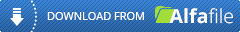## GraphPad Prism 7.00 Build 159GraphPad Prism 7.00 Build 159 | 17.2 MB

GraphPad Prism combines scientific graphing, comprehensive curve fitting (nonlinear regression), understandable statistics, and data organization. GraphPad Prism was originally designed for experimental biologists in medical schools and drug companies, especially those in pharmacology and physiology. Prism is now used much more broadly by all kinds of biologists, as well as social and physical scientists. More than 200,000 scientists in over 110 countries rely on Prism to analyze, graph and present their scientific data. It is also widely used by undergraduate and graduate students.

Statistical comparisons
-Paired or unpaired t tests. Reports P values and confidence intervals.
-Nonparametric Mann-Whitney test, including confidence interval of difference of medians.
-Kolmogorov-Smirnov test.
-Wilcoxon test with confidence interval of median.
-Perform many t tests at once, using False Discover Rate to choose which comparisons are discoveries to study further.
-Ordinary or repeated measures one-way ANOVA followed by the Tukey, Newman-Keuls, Dunnett, Bonferroni or Holm-Sidak multiple comparison tests, the post-test for trend, or Fisher's Least Significant tests.
-Many multiple comparisons test are accompanied by confidence intervals and multiplicity adjusted P values.
-Greenhouse-Geisser correction so repeated measures one-way ANOVA does not have to assume sphericity. When this is chosen, multiple comparison tests also do not assume sphericity.
-Kruskal-Wallis or Friedman nonparametric one-way ANOVA with Dunn's post test.
-Fisher's exact test or the chi-square test. Calculate the relative risk and odds ratio with confidence intervals.
-Two-way ANOVA, even with missing values with some post tests.
-Two-way ANOVA, with repeated measures in one or both factors. Tukey, Newman-Keuls, Dunnett, Bonferron, Holm-Sidak, or Fishers LSD multiple comparisons testing main and simple effects.
-Kaplan-Meier survival analysis. Compare curves with the log-rank test (including test for trend).

Column statistics
-Calculate min, max, quartiles, mean, SD, SEM, CI, CV,
-Mean or geometric mean with confidence intervals.
-Frequency distributions (bin to histogram), including cumulative histograms.
-Normality testing by three methods.
-One sample t test or Wilcoxon test to compare the column mean (or median) with a theoretical value.
-Skewness and Kurtosis.
-Identify outliers using Grubbs or ROUT method.

Linear regression and correlation
-Calculate slope and intercept with confidence intervals.
-Force the regression line through a specified point.
-Fit to replicate Y values or mean Y.
-Test for departure from linearity with a runs test.
-Calculate and graph residuals.
-Compare slopes and intercepts of two or more regression lines.
-Interpolate new points along the standard curve.
-Pearson or Spearman (nonparametric) correlation.

Kod:
`````
`http://alfafile.net/file/hRXi/3klku.GraphPad.Prism.7.00.Build.159.rar`